# Pandas How to replace values based on Conditions

Using these methods either you can replace a single cell or all the values of a row and column in a dataframe based on conditions .

Dataframe:

``````import pandas as pd
import numpy as np

df = pd.DataFrame({'Date' : ['11/8/2011', '11/9/2011', '11/10/2011',
'11/11/2011', '11/12/2011'],
'Event' : ['Dance', 'Painting', 'Dance', 'Dance', 'Painting']})

df
``````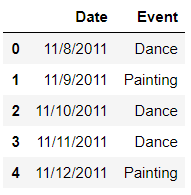## Using loc for Replace

Replace all the Dance in Column Event with Hip-Hop

``````df.loc[(df.Event == 'Dance'),'Event']='Hip-Hop'
df
``````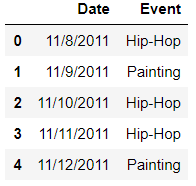## Using numpy where

Replace all Paintings in Column Event with Art

``````df['Event'] = np.where((df.Event == 'Painting'),'Art',df.Event)
df
``````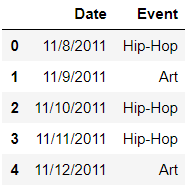Replace all the Hip-Hop in Column Event with Jazz

``````df['Event'].mask(df['Event'] == 'Hip-Hop', 'Jazz', inplace=True)
``````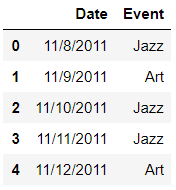## Using df where

Replace all Art in Column Event with Theater

``````m = df.Event == 'Art'
df.where(~m,other='Theater')
``````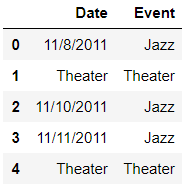## Create a new Dataframe

``````df = pd.DataFrame([[1.4, 8], [1.2, 5], [0.3, 10]],
index=['China', 'India', 'USA'],
columns=['Population(B)', 'Economy'])
``````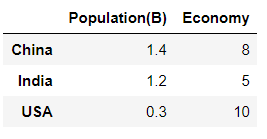## Set value for an entire row

``````df.loc['India'] = 10
df
``````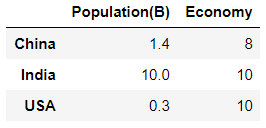## Set value for an entire column

``````df.loc[:, 'Economy'] = 30
df
``````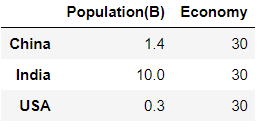## Set value for rows matching condition

``````df.loc[df['Population(B)'] < 1] = 0
df
``````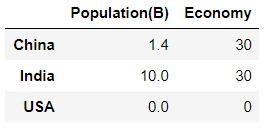Categories:

Updated: